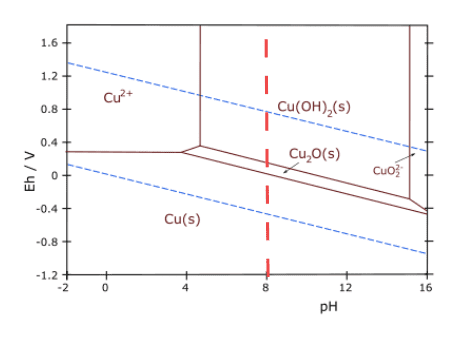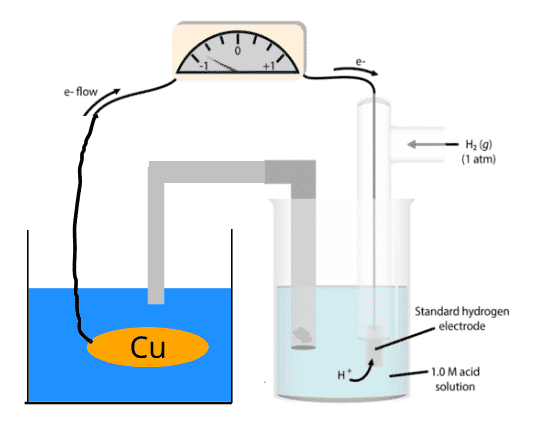# Using Pourbaix diagrams to calculate corrosion in water

• Chemistry
wnvl2
Homework Statement:
Which of the metals Al, Cu, Fe, Ag, Pb and Au can (thermodynamically) corrode in water that is Slightly acidic (pH = 6) (AI, Cu, Fe, Ag, Pb) Slightly basic (pH = 8) (AI, Cu, Fe, Pb) Very acidic (pH = 1) (AI, Cu, Fe, Ag, Pb) Very basic (pH = 14) (AI, (Cu), Fe, Pb) . .
Relevant Equations:
Use Pourbaix diagramLet us solve it for Cu at pH =8. I found this Pourbaix diagram. What is the electrochemical potential I should use to check that Cu is there the stabel form. Should I check for all values of E between the blue lines at pH = 8? How can I measure the E value in reality?

Last edited by a moderator:

Gold Member
In the case of pH 8, your task is pretty easy. But in general, the Pourbaix diagram will give you the potentials/pH’s over which different forms of a metal are stable in water (usually).

As for what the potential is in an experimental situation, it’s either chosen by you when you apply a voltage or it can be measured straightforwardly by a potentiometer.

•Astronuc and wnvl2
wnvl2
If I am right, I can connect the copper to a SHE, measure the voltage and that is the E I have to use in the Pourbaix diagram. As the E is not specified in the original question, I can not give a unique answer.Staff Emeritus
Gold Member
As the E is not specified in the original question, I can not give a unique answer.
You should try to answer the question nonetheless.

I'll try to frame the question somewhat more clearly. For your example, copper at pH=8, over what range of potential will it corrode?

•Astronuc
Mentor
What it is that is present everywhere and makes metals corrode?

wnvl2
Oxygen?

Mentor
Combined with pH it should give you some idea about the potentials involved.

Not that they are ignored on the diagram.

wnvl2
Does that mean that if the copper is in a water solution in abscence of oxygen that the copper will not corrode?

Gold Member
Does that mean that if the copper is in a water solution in abscence of oxygen that the copper will not corrode?
I’m not sure what borek is getting at, but this is definitely not true. Did you try answering my question in post 5?

wnvl2
Above 0.1V there is oxidation. Below 0.1V cupper is stable.

•TeethWhitener
•